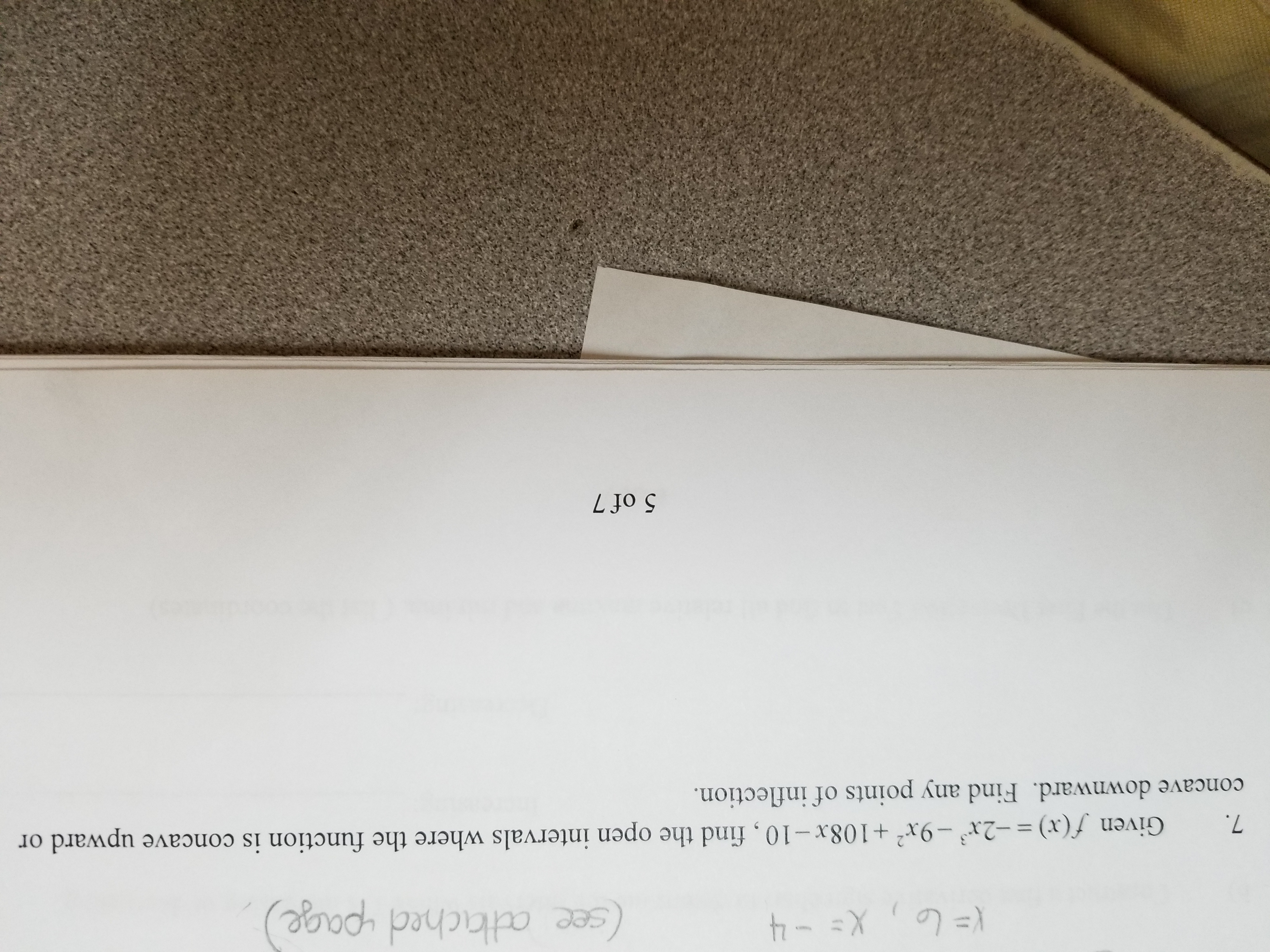(see athchad -page7. Given)x+108x-10, find the open intervals where the function is concave upward orconcave downward. Find any points of inflection) =-2x3-9x2 + 108x-10 , find the open intervals where the function is concave upward or5 of 7

Question

How do I find open intervals.  I am attaching a problemhelp_outlineImage Transcriptionclose(see athchad -page 7. Given)x+108x-10, find the open intervals where the function is concave upward or concave downward. Find any points of inflection ) =-2x3-9x2 + 108x-10 , find the open intervals where the function is concave upward or 5 of 7 fullscreen
Step 1

We have to find inflection point and open interval of concave up and concave down of f(x) where f(x) is given by:

Step 2

We will use power rule of derivative to find f''(x) .

Power rule of derivative is given by

Step 3

Inflection point of f(x) is where f''(x)=0 so with the help of power rule of derivative(use two times t...

Want to see the full answer?

See Solution

Want to see this answer and more?

Our solutions are written by experts, many with advanced degrees, and available 24/7

See Solution
Tagged in

Calculus